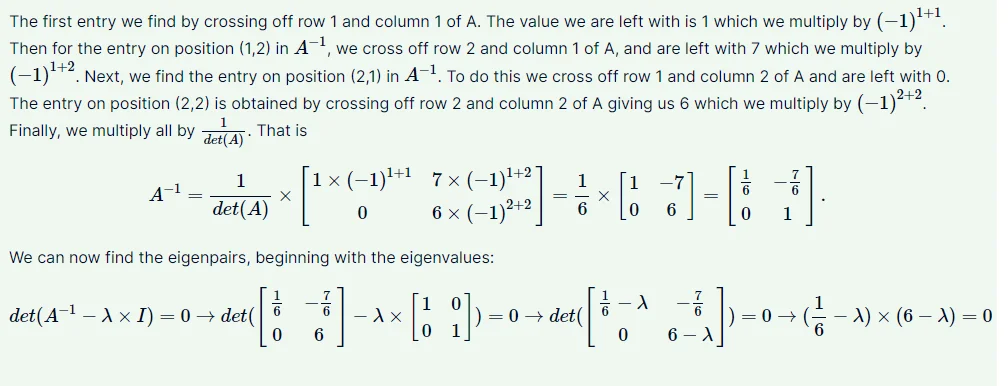13 Dec 2022

Posted on:

28 Nov 2022

2

# Errors in the final exams

Hello,
I would like to report some issues about the final exam.
In the question 4, the correct matrix D isn't in the list, in the position (1,0) should be zero.
Also I couldn't get the correct answer for between-scatter matrix calculation (the maximum value). In my calculations it is

``````5940.8
``````

Which is not presented in the answer options.
Maybe you should double check it, it would be great addition, if you insert in the explanation section - the correct code lines to obtain the same result as you have.

Thank you!

Instructor
Posted on:

29 Nov 2022

0

Hi Tatiana,
thanks for reaching out and for the feedback! I'll revise question 4 to include the correct eigenvalues.
The code you'd need to obtain the between scatter matrix is the following:

``````overall_mean=np.mean(X_std,axis=0)

S_b=np.zeros((5,5))
for i,mean_vec in enumerate(mean_vectors):
n=X_std[y==i+1,:].shape

mean_vec=mean_vec.reshape(5,1)
overall_mean=overall_mean.reshape(5,1)
S_b+=n*(mean_vec-overall_mean).dot((mean_vec-overall_mean).T)

print('Between class scatter matrix:\n',round(S_b.max(),1))
``````

You'd need to create the X and y matrix and column vector first, and then standardize X with a standardscaler to obtain X_std.
Let me know if you have any furhter questions.

Best,
365 Eli

Super learner
Posted on:

12 Dec 2022

1

Hi,
I guess you meant question 5 where we should calculate matrix D square.
In description matrix D is 2x2 - [[1 0],[0 10]], main diagonal is eigenvalue of matrix A - 1 and 10, outside diagonal are zeros. so D square had to be [[1 0],[0 100]] as Tatiana wrote.
Also could you please add better explanation for answers 4 and 5 because I could not understood on the beginning what I did wrong?

Super learner
Posted on:

13 Dec 2022

0

inverted matrix A was correctly calculated but then when applied to formula of calculation eigenvalues position (2,2) was replaced with 6 instead of 1 so entire result was incorrect for second rootPosted on:

13 Dec 2022

0

Yes, exactly that question, thank you, Vladyslav!

Posted on:

13 Dec 2022

0

Elitsa, thank you for the answer!
I reviewed the code, could we please discuss this line?

``````<span class="colour" style="color: rgb(116, 129, 144);"><b><span class="colour" style="color: unset;">**<span class="colour" style="color: unset;">**<span class="colour" style="color: unset;">**<span class="colour" style="color: unset;">**for**</span>**</span>**</span>**</span></b> i,mean\_vec <b><span class="colour" style="color: unset;">**<span class="colour" style="color: unset;">**<span class="colour" style="color: unset;">**<span class="colour" style="color: unset;">**in**</span>**</span>**</span>**</span></b> enumerate(mean\_vectors):</span></b> enumerate(mean_vectors):
</span>        n=X_std[y==i+1,:].shape
``````

In this code i takes values 0 and 1,  so in the brackets you check the conditions in which y takes values 1 and 2, but it is not correct as the labels for classes are 0 and 1, so in my code it is

``````for i,mean_vec in enumerate(mean_vectors):
n=X_std[y==i,:].shape
``````

And the result matrix is different. Could you please check this piece of code and explain why are you incrementing i in the brackets?